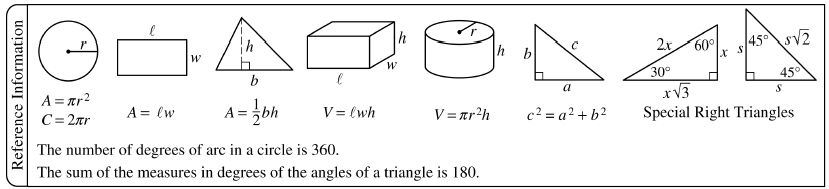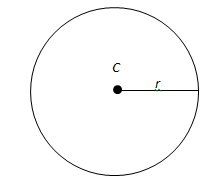# Know the SAT Math Formula Sheet! (Part 1 of 8)Hello Everyone! I’m SAT Perfect Scorer Joey Gu. After taking the Test Masters SAT & PSAT course and getting a Perfect Score on the SAT, I was offered a summer internship position with Test Masters. Obviously, I accepted their offer. In between data entry and other “special projects,” I’ve been asked to share some of my SAT knowledge and experience with everyone in this blog-o-sphere.

The SAT Math formula sheet can seem imposing. It is the first thing you see at the beginning of every SAT & PSAT Math section, and if you are unfamiliar with the applications or concepts behind each of the formulas shown, its presence can turn an advantage into a psychological disadvantage. For this reason, I have been asked to do an SAT Math series in which I use these formulas to answer different questions. I hope you all enjoy it, and please feel free to comment or email me any questions!Given that the circumference of circle C is 18π, what is the area of circle C?This problem uses the first diagram in the “Reference Information” box of the SAT Math Section. The diagram shows a circle with radius r. Below the circle, there are two formulas, both of which apply to circles only. The first formula is A = πr2, which is used to find the area within a circle. The second formula is C = 2πr, which is used to find the perimeter of the circle, a.k.a. the circumference. In this problem, the circumference is given. Since we can only start with what we are given, let’s begin with the circumference formula.

C = 2πr

The question states that the circumference is equal to 18π. Therefore, our next step is to substitute 18π into the formula.

18π = 2πr

Since r is the only variable left, we should isolate and solve for r. To do this, divide both sides by 2π.

9 = r

We now know that the radius of the circle is 9. Because we know the radius, we can also solve for the area, which is what the question is asking for.

A = πr2

= π(9)2

= 81π

Therefore, the area of circle C is 81π.

Return next week as I continue College Compass’ “Know the SAT Math Formula Sheet!” series.

By Joey Gu

### You Might Also Like

READ  ACT: What is Test Information Release?

1.Mercedes R. says:
1.Calvin says: